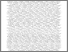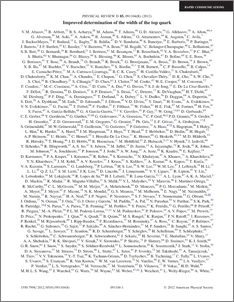# An improved determination of the width of the top quark

Collaboration, D0 and Bertram, Iain and Borissov, Guennadi and Fox, Harald and Ross, Anthony and Williams, Mark and Ratoff, Peter (2012) An improved determination of the width of the top quark. Physical Review D, 85 (9). ISSN 1550-7998Preview
PDF
PhysRevD.85.091104.pdf - Published Version

## Abstract

We present an improved determination of the total width of the top quark, $\Gamma_t$, using 5.4 fb$^{-1}$ of integrated luminosity collected by the D0 Collaboration at the Tevatron $p\bar{p}$ Collider. The total width $\Gamma_t$ is extracted from the partial decay width $\Gamma(t\to Wb)$ and the branching fraction $\mathcal{B}(t\to Wb)$. $\Gamma(t\to Wb)$ is obtained from the $t$-channel single top quark production cross section and $\mathcal{B}(t\to Wb)$ is measured in $t\bar{t}$ events. For a top mass of $172.5\;\rm GeV$, the resulting width is $\Gamma_t = 2.00^{+0.47}_{-0.43}$ GeV. This translates to a top-quark lifetime of $\tau_t = (3.29^{+0.90}_{-0.63})\times10^{-25}$ s. We also extract an improved direct limit on the CKM matrix element $0.81$|V_{tb}| 0.59\$ for a high mass fourth generation bottom quark assuming unitarity of the fourth generation quark mixing matrix.

Item Type:
Journal Article
Journal or Publication Title:
Physical Review D